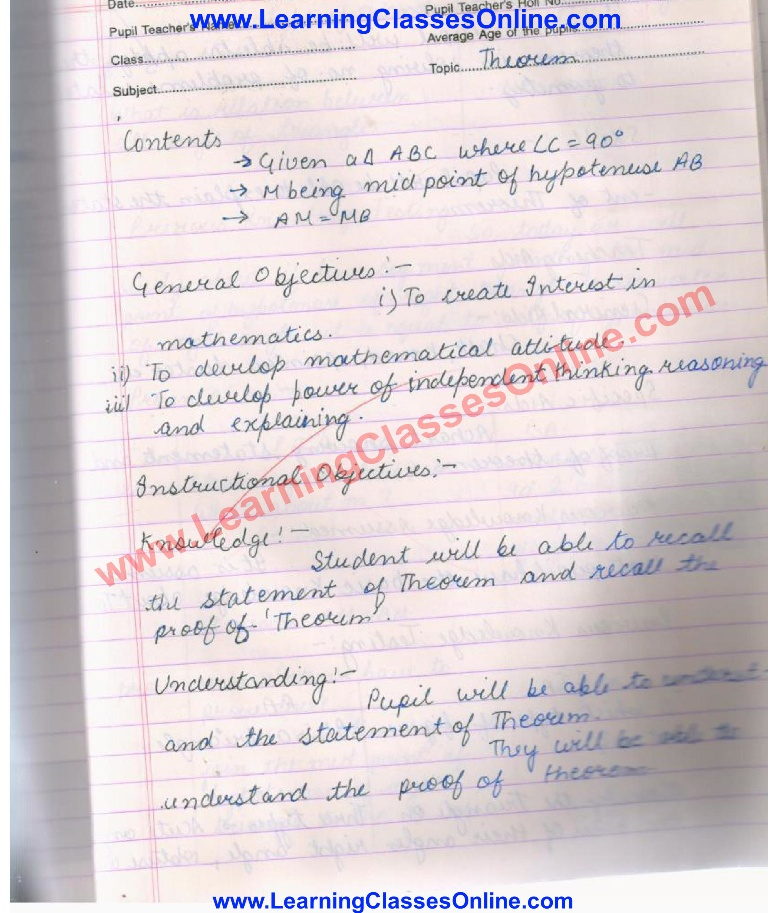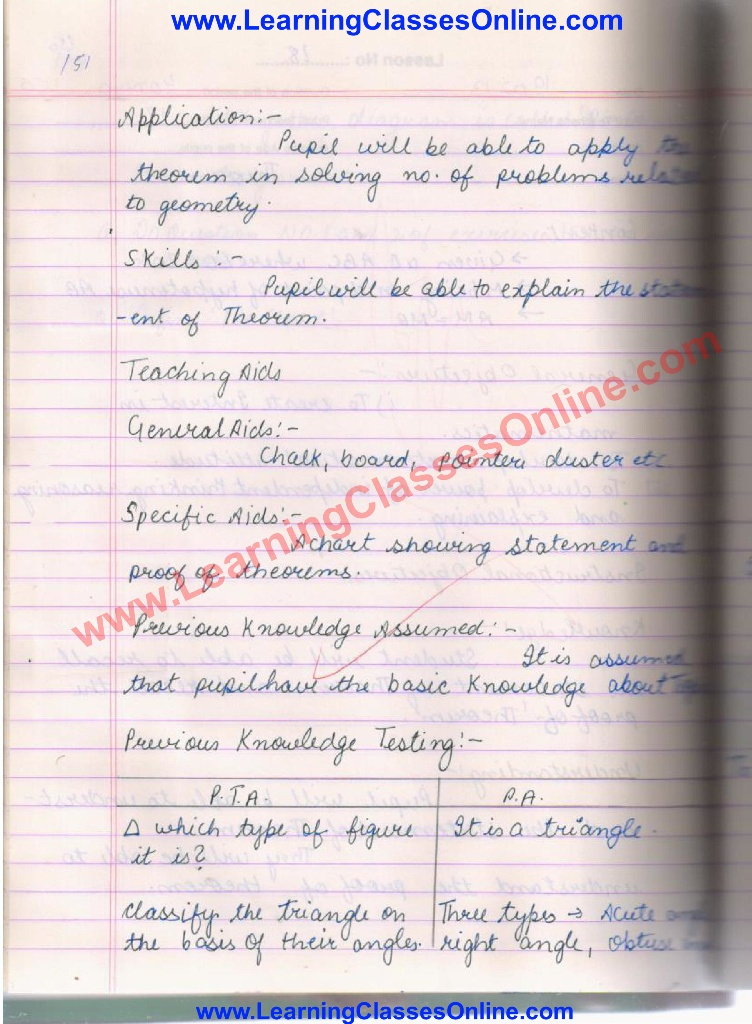Theorem Lesson Plan

# Theorem Lesson Plan

Math Lesson Plan on Theorem right angle triangle if line segment joining the midpoint of the hypotenuse to the vertex of a right angle then this line is equal to half of the hypotenuse.Use of SAS ( side angle side congruence), use of CPCT( corresponding parts of congruent triangles)

• Duration: 40 minutes
• Subject: Mathematics
• Topic: Theorem
• Lesson Plan Type: Real School Teaching and Practice
• Language: English

Note: The Mathematics Lesson Plan given below is just an example.You can change the name, class, course, date etc. according to your need.